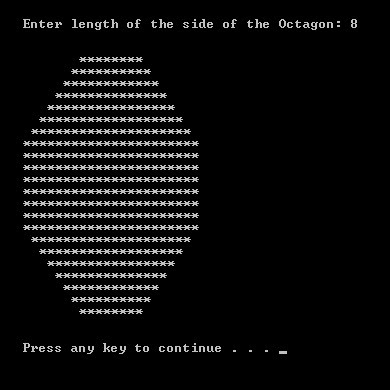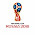Printing Octagon | C++ Program to Print Patterns

Printing Octagon | C++ Program to Print Patterns#include <iostream>
using namespace std;

int main()
{
int side,line=1,star=1,space=1,no_of_spaces,no_of_stars;

cout << "Enter length of the side of the Octagon: ";
cin >> side;

cout << "\n\n";

no_of_spaces = side;
no_of_stars = side;

for (line = 1; line <= side; line++){

for (space = 1; space<no_of_spaces; space++)
cout << " ";

for (star = 1; star <= no_of_stars; star++)
cout << "*";

no_of_spaces--;
no_of_stars += 2;

cout << endl;
}

no_of_stars -= 2;

for (line = 1; line<side; line++){

for (star = 1; star <= no_of_stars; star++)
cout << "*";

cout << endl;
}

no_of_spaces = 1;
no_of_stars -= 2;

for (line = 1; line<side; line++){

for (space = 1; space <= no_of_spaces; space++)
cout << " ";

for (star = 1; star <= no_of_stars; star++)
cout << "*";

no_of_spaces++;
no_of_stars -= 2;

cout << endl;
}

cout << "\n\n";
return 0;
}

1.2.